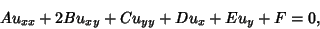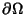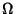## Elliptic Partial Differential Equation

A second-order Partial Differential Equation, i.e., one of the form(1)

is called elliptic if the Matrix(2)

is Positive Definite. Laplace's Equation and Poisson's Equation are examples of elliptic partial differential equations. For an elliptic partial differential equation, Boundary Conditions are used to give the constrainton, where(3)

holds in.

See also Hyperbolic Partial Differential Equation, Parabolic Partial Differential Equation, Partial Differential Equation